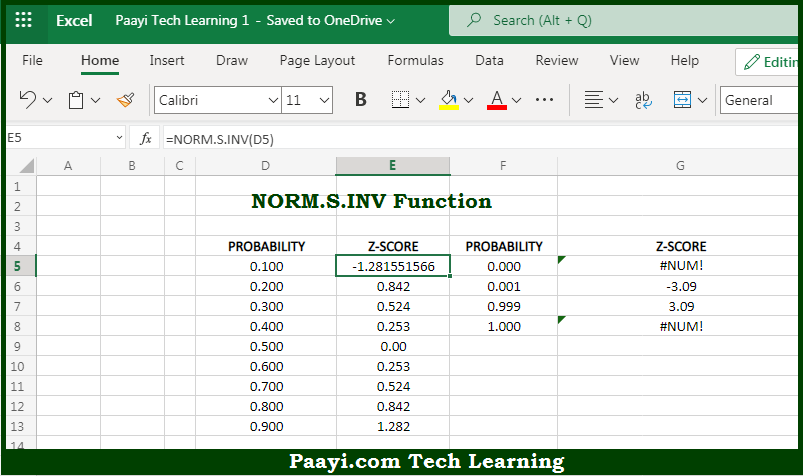# Learn How to Use Microsoft Excel NORM.S.INV Function

Written by | 0 Comments | 701 Views

In this article, you will learn how to use Microsoft Excel NORM.S.INV function and its prime function in Microsoft Excel. You will also get to know the Microsoft Excel NORM.S.INV function return value and syntax with the help of some examples.

Microsoft Excel NORM.S.INV Function

The main purpose of the Microsoft Excel NORM.S.INV function is to get the inverse of standard normal cumulative distribution. That implies, with the help of NORM.S.INV function you can able to return the inverse of the standard normal cumulative distribution. So, with the help of NORM.S.INV function, you can able to get the inverse of standard normal cumulative distribution.

Return Value of NORM.S.INV Function

The return value will be the threshold value of the probability.

Syntax of NORM.S.INV Function

=NORM.S.INV(probability)

Where the arguments:

• probability: This is the probability corresponding to the standard normal distribution.

How to Use Microsoft Excel NORM.S.INV Function?So we know that, Microsoft Excel NORM.S.INV function you can able to get the inverse of standard normal cumulative distribution. That implies, with the help of NORM.S.INV function you can able to return the inverse of the standard normal cumulative distribution. So, with the help of NORM.S.INV function, you can able to get the inverse of standard normal cumulative distribution.